Statistical computations in Maple combine the ease of working in a high-level, interactive environment with a very large and powerful set of algorithms. Large data sets can be handled efficiently with 35 built-in statistical distributions, sampling, estimations, data smoothing, hypothesis testing, and visualization algorithms. In addition, integration with the Maple symbolic engine means that you can easily specify custom distributions by combining existing distributions or simply by giving a formula for the probability or cumulative distribution function.

Maple 16 includes significant enhancements to the Statistics package. These enhancements include:

• Discrete distributions, which are important in many areas from game theory to algorithm analysis, are significantly enhanced, with support for non-integer values as well as sampling of custom discrete distributions.
• Maximum likelihood estimation now allows for multiple parameters and is significantly faster.
• Improved support for matrix data sets makes it is easier to split data into subsets based on particular criteria, enhancing your ability to analyze data and identify patterns.
• Statistical visualization is easier than ever before. In addition to the new Live Data Plots, enhancements like variable bin-width histograms and new options for pie charts provide you with extra control over how data is presented.Details and ExamplesDiscrete distributions with noninteger values
New in Maple 16, Maple supports discrete distributions that can have noninteger values.

Example: At the market
At a market stall, a vendor charges \$5 for taking part in a game of chance. If you participate, you will receive the contents of one of four envelopes filled with small change, each with probability 1/4. The values of the envelopes are \$8.24, \$3.77, \$3.91, and \$0.16.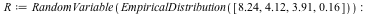We can find the expected amount of money we will receive as=. This sounds like a fairly unappealing deal.

Suppose furthermore that we are interested in buying peanuts with this money. Because of overhead, buying a few peanuts is more expensive per peanut than if you buy a lot of them. Our peanut supplier will sell usgrams of peanuts for \$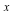(for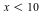), so for \$5, we can get 250g of peanuts. The expected weight of peanuts we will get if we participate in the game is:=, or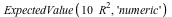=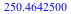.

Since squaring so strongly benefits the highest outcome, \$8.24, the expected payout in peanuts if we take part in the game, is essentially equal to the payout without taking part in the game.

When the probabilities for different outcomes are not all the same (or all small multiples of a single value), we can use the new probabilities option to EmpiricalDistribution. In a more refined model, the weight or volume of the envelopes might influence how likely each one is to be picked. For example, suppose the probabilities are as follows:where the top row gives the values in dollars as before and the bottom row gives the probabilities. (This information is tied to the variableusing the data table feature.) Doing the same computations as above, we now see: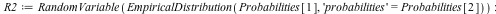=. The expected outcome is higher, but still falls short of the price. However, the expected weight of peanuts is now=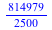or=.

We now see that the payout in peanuts is better if we take part in the game!

Custom distributions
EmpiricalDistribution can be used for all discrete distributions that can assume only finitely many values. All the discrete distributions that can assume infinitely many values that are built into Maple only support integer values. Therefore, to use a discrete distribution that can assume infinitely many values, some or all of which are not integers, we need to define this distribution ourselves, using the custom distribution feature of the Statistics package.

Consider the distribution that can assume all negative powers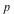of 2, each with probability. That is, the corresponding random variable iswith probability,with probability, and so on. This distribution is represented as follows: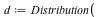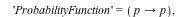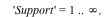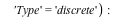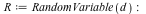The DiscreteValueMap and Support properties determine what values the probability distribution can assume; the ProbabilityFunction determines the probability that that value is assumed. For more details, see the DiscreteValueMap help page. We can now compute that=, for example, or=, or=.

Another distribution can be obtained by taking the probabilities, for(a modified negative binomial distribution) and associating the
valuewith theth probability. This is defined as follows: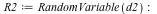Again, we can compute such quantities as=and=.

Sampling custom discrete distributions

In previous versions, Maple did not support sampling of custom discrete distributions. This feature was added to Maple 16.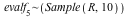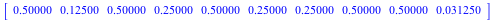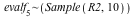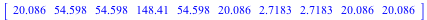Parameter estimation is more efficient and handles more cases
Maple 16 has much more efficient and robust routines for doing maximum likelihood parameter estimation for many distributions. The following example was sped up by a factor of about 10.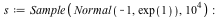memory used=2.35MiB, alloc change=0 bytes, cpu time=78.00ms, real time=97.00msMaple can now also estimate multiple parameters at the same time using maximum likelihood estimation.memory used=1.14MiB, alloc change=0 bytes, cpu time=31.00ms, real time=69.00msMatrix data sets

The Statistics package in Maple 16 has been updated to better handle Matrix data sets. In previous releases, there were instances where the Statistics package did not always accept Matrix data types. In Maple 16, the commands in the Statistics package have been updated to work with Matrix data sets. These commands work on each column of its input Matrix separately. In addition, Maple 16 now allows you to split your data into submatrices based on the value of one column. As a result you are now able to organize and present your data in different configurations in order to better observe particular trends.

As an example, assume the data below represents some housing data. The first column has the number of bedrooms, the second column has the number of square feet, the third has the price in dollars. This data table corresponds to the variable.Using the SplitByColumn command we can easily rearrange the data in terms of the number of bedrooms:If we want to know the average area and price for the three bedroom houses, we can find that as follows: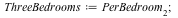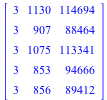We see that the average three bedroom house has an area of about 960 square feet and costs just over \$100100.

Statistical Visualization

Live data plots

A new palette in Maple 16 makes it easy to create and customize statistical plots, including area charts, histograms, pie charts, and scatter plots. For more information, see Live Data Plots in Maple 16.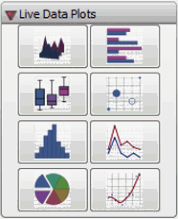Variable-width histograms

Sometimes a phenomenon that lends itself to display using a histogram changes rapidly in a certain region and not so rapidly in a different region. In the region where the phenomenon changes rapidly, you would like to show a very fine-grained histogram, but elsewhere that would be overkill and be distracting. In this case, you can use the variable-width bins feature for histograms, new in Maple 16.

For example, suppose you have data that can come from one of two processes: either from a Beta distribution with parameters 3 and 2.5, or from a normal distribution with mean -2 and standard deviation 5. We have 10000 elements from each.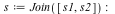The default histogram is too coarse:But a histogram with a much finer bin width shows too many empty bins: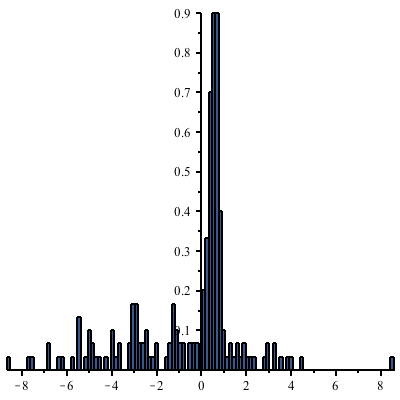The following command uses wider bins where there are fewer points. (The heights of the rectangles are proportional to the density of points in the given bin, and the total area of all rectangles is 1.)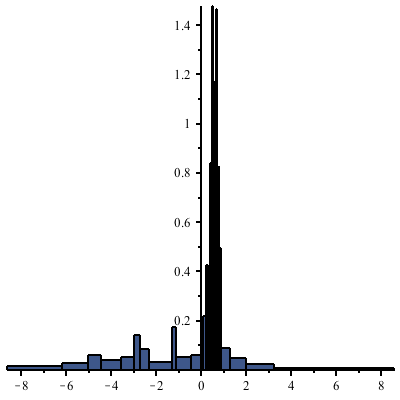ScatterPlot3D

• The ScatterPlot3D command provides functionality to plot a surface from an mx3 Array or Matrix representing points in three-dimensional space. The surface is a smoothed approximation, generated using the lowess algorithm. Considering each row of the data Matrix as a point in x;-y;-z; space then the first two entries of each row represent a point on the x;-y; plane (independent data) while the third entry of each row represents the z;-coordinate (dependent data).
• The data in the first two entries of each row does not need to form a regular grid in the (-) plane.
• The following example constructs data by adding noise to a function (-value) in the first two (and) dimensions.

> X := Sample(Uniform(-50,50),175):
> Y := Sample(Uniform(-50,50),175):
> Zerror := Sample(Normal(0,100),175):
> Z := Array(1..175,(i)->-(sin(Y[i]/20)*(X[i]-6)^2+(Y[i]-7)^2+Zerror[i])):
> XYZ := Matrix([[X],[Y],[Z]],datatype=float)^%T;> ScatterPlot3D(XYZ, axes=box, orientation=[20,0,0]);> ScatterPlot3D(XYZ, lowess, grid=[25,25], axes=box, orientation=[20,70,0]);Pie chart improvements

In Maple 16, you can now create three-dimensional pie charts and annular pie charts.

Additional improvements include new default coloring of pie charts. When you specify ranges for pie chart colors, the two range endpoint colors are plotted opposite each other in the pie chart. The color gradient for the pie chart changes clockwise. The colors in the pie chart are kept within the same hue.

In addition, labels of pie slices are automatically colored for better contrast.

> dataset := ["A" = 5, "B" = 4, "C" = 3, "D" = 2, "E" = 3, "F" = 4, "G" = 5]:
> Statistics:-PieChart(dataset, color = "CornflowerBlue" .. "DarkBlue", annular = true, render3d = true);How to Proceed: Pricing & Purchase Evaluate Upgrade Get Price Quote Buy Online